# How to LOOKUP Value in Excel with Multiple Criteria – 6 Methods

You can easily look up a value in a table using one criterion. Here you will apply the VLOOKUP formula. But at times, you may need to use more than one criterion. This may seem like a pretty hard thing to achieve in Excel but it is not the case. Excel offers several functions you can use.

Here, we discuss step-by-step how to look up in Excel with multiple criteria.

## Using SUMPRODUCT Function to lookup in Excel with multiple criteria

You can use two criteria to return a value in a cell by using the SUMPRODUCT function. Your function will include the following:

`=SUMPRODUCT ((criteria 1)*(criteria2)*(cells to sum))`

You can also this Excel function if you have more than two criteria. You will add the other criteria into the formula to get your results.

For example, in the data below, we want to look up the product and color and then return the price. Therefore the multiple criteria, in this case, are product and color.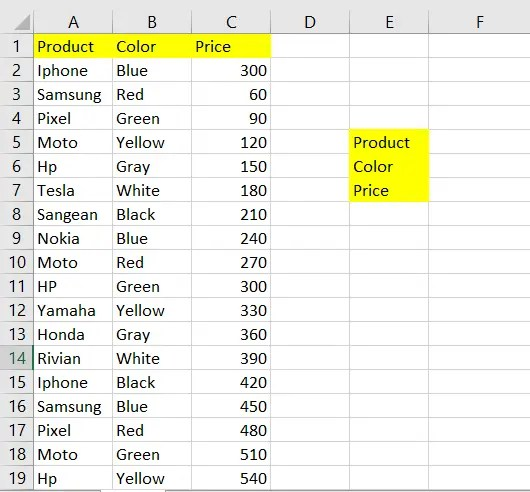As you can see from the image above, we have created a small section with multiple criteria and prices. We will use the sum product formula to display the price of the multiple criteria in cell F7.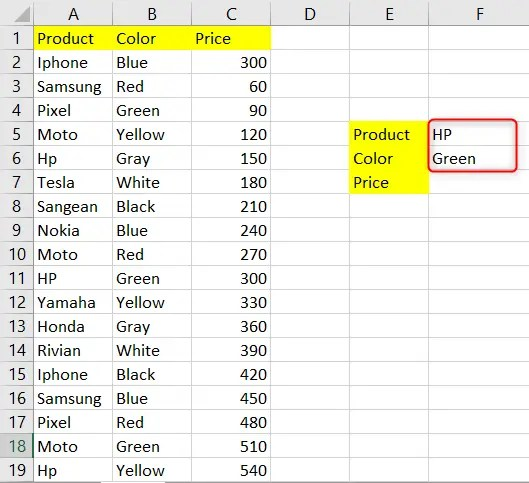The first thing you ought to do is list your multiple criteria according to the labels in F5 and F6, respectively.

Next step, you need to enter the following formula

`=SUMPRODUCT((A2:A19=F5)*(B2:B19=F6)*(C2:C19))`

The formula above looks for the value F5 from the range A2:A19. In addition, it also looks for F6 from the range B2:B19. After finding both values, the formula looks for the missing value in the range C2:C19 that you provided in F7. Take a look at the screenshot below and find out how it returns a value after searching multiple criteria.The formula returns 300, which is the price corresponding to HP and Green. From the List, there is also HP which corresponds with Gray, and HP, which corresponds with Yellow. But since they do not match the multiple criteria that we are searching the formula ignores them.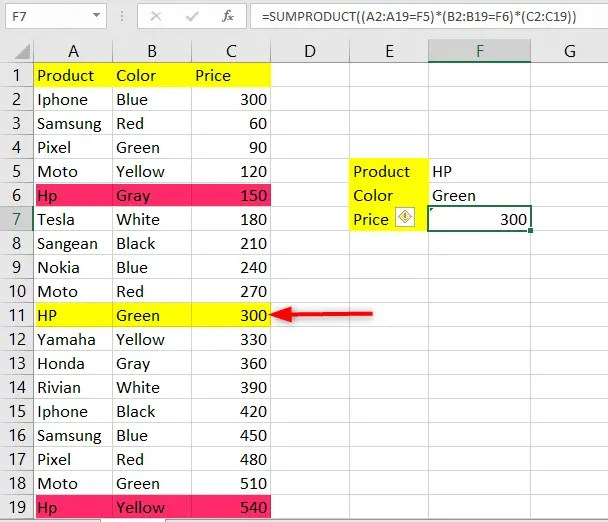The sumproduct function only works well when you are looking up values using multiple criteria.  Thus you cannot search for text using the sum product. It will result in a value error, as you can see in the screenshot below.## Using SUMIFS

SUMIFS formula can also look for values based on multiple criteria.  Use the formula below.

`=SUMIFS(C2:C17,A2:A17,F5,B2:B17,F6)`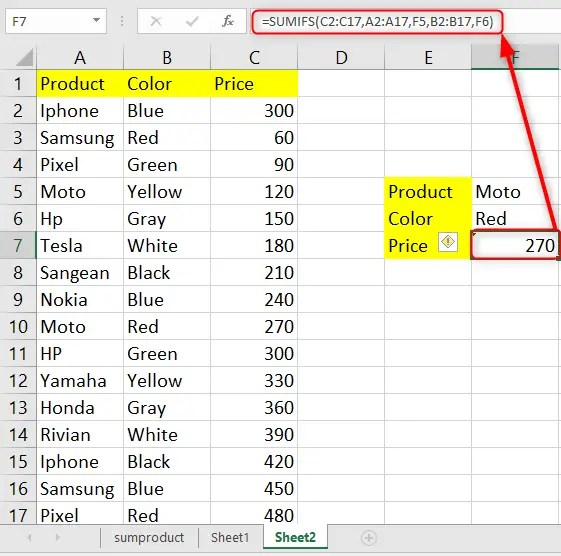How to filter in Excel greater than

How to sum in Excel between sheets

## Using the SUM function

Enter the following formula in cell F7

`=SUM((A2:A17=F5)*(B2:B17=F6)*(C2:C17))`## Using INDEX/MATCH functions to lookup in Excel

You can use the INDEX and Match functions when doing a multiple criteria Excel Lookup. These two functions serve different purposes. The INDEX function returns a value from a specific cell in a list within your worksheet. On the other hand, the MATCH function will find the location of an item within a list.

The INDEX function has three arguments, namely:

Array: which represents where the array is

Row_num: represents the row with the value you want to be returned.

[column_num]: shows the column with the value you want to be returned.

The MATCH formula consists of 3 arguments namely:

lookup_value that looks for the value you wish to find in the  array

lookup_array: represents where the lookup array is.

[match_type] finds an exact match or a closer match.

The INDEX-MATCH functions will only return the result of what you are specifically looking for. You can add the FILTER function to get all the values that will satisfy the given criteria.

Your formula for index and match functions will look like this:

=INDEX (lookup_range, MATCH (1, INDEX ((criteria1 =range1)*(criteria2=range2)*(criteria N=range N), 0, 1), 0))

Whereby:

The lookup_range: represents the range from which you want to retrieve the value.

Criteria1, Criteria2, and Criteria N: represent the criteria you want to match in range 1, range 2, and range N.

Rtange1, Range2, and Range N: represent the ranges in which you will match your respective criteria.Note that this is an array formula; therefore, you need to hold Ctrl+Shift+Enter.

## Using LOOKUP function

Enter the following formula

`=LOOKUP(2,1/(A2:A17=F5)/(B2:B17=F6),(C2:C17))`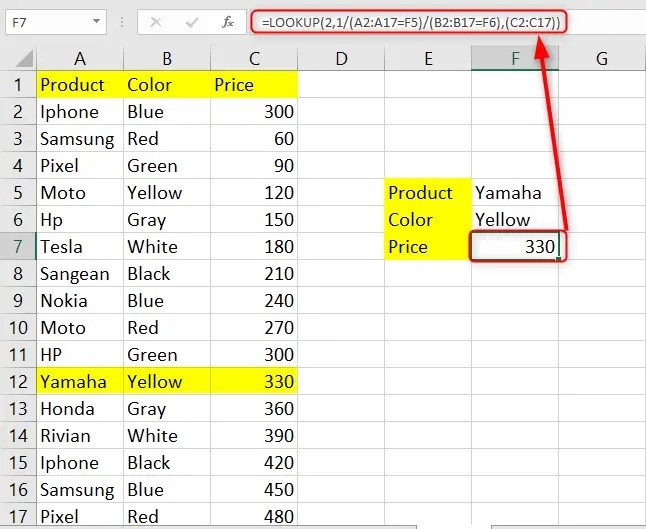Note that you can change the criteria, and the price will adjust automatically.

How to lookup value based on multiple criteria practice template

## Using the FILTER function to lookup in Excel 365

The FILTER function only works in Office 365. You can use this function to return the desired results from a range based on your criteria.

The FILTER function has 3 arguments that include:

The array, includes, and if_empty arguments. Your formula will look like this:

=FILTER (array, include, [if_empty])

Enter the following formula and press enter

`=FILTER(C2:C10,(B2:B10=D16)*(A2:A10=D15))`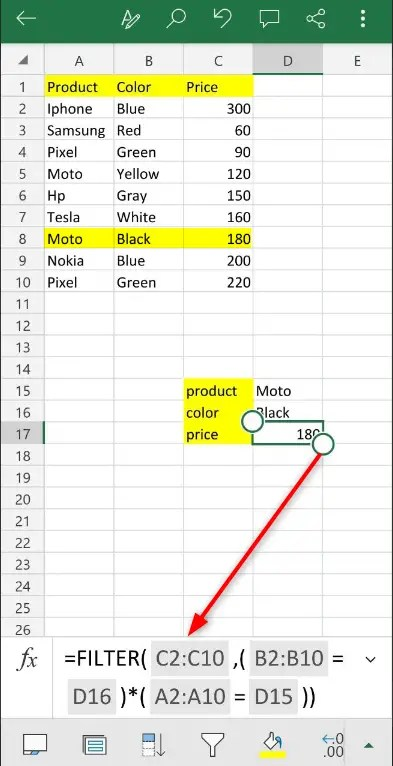As you can see from the screenshot above the FILTER function has filtered the the price using product and color as the multiple criteria. Note that the =FILTER function is only available on Microsoft 365.